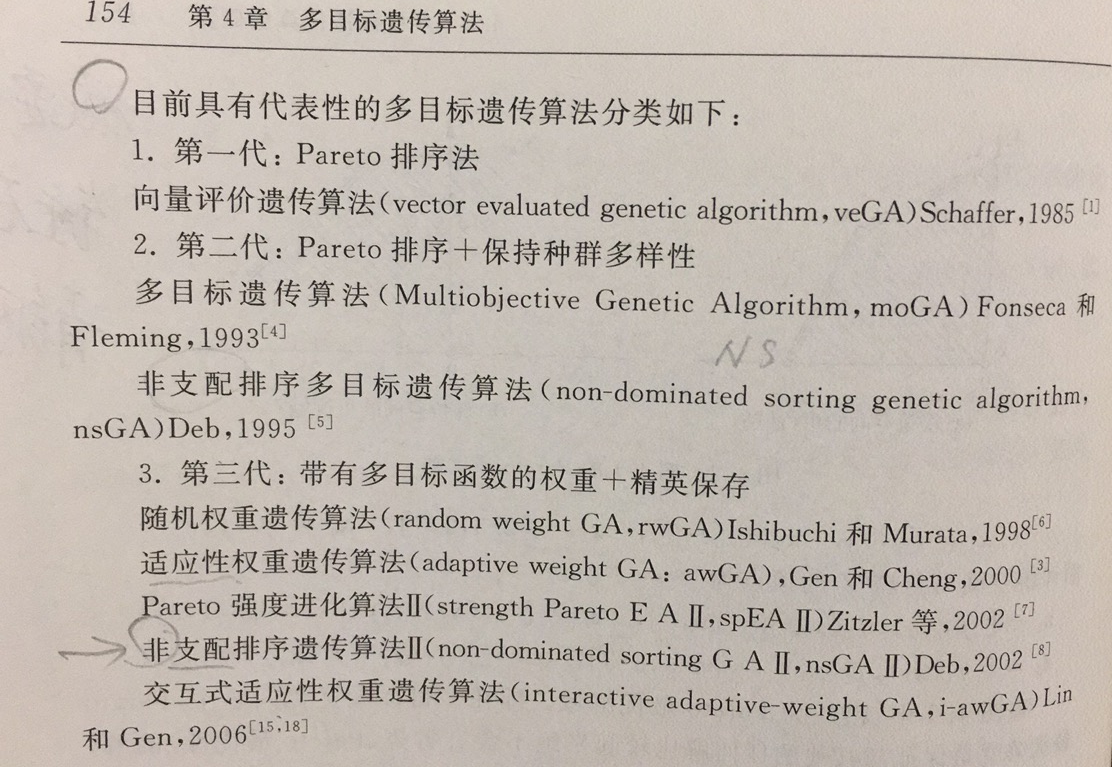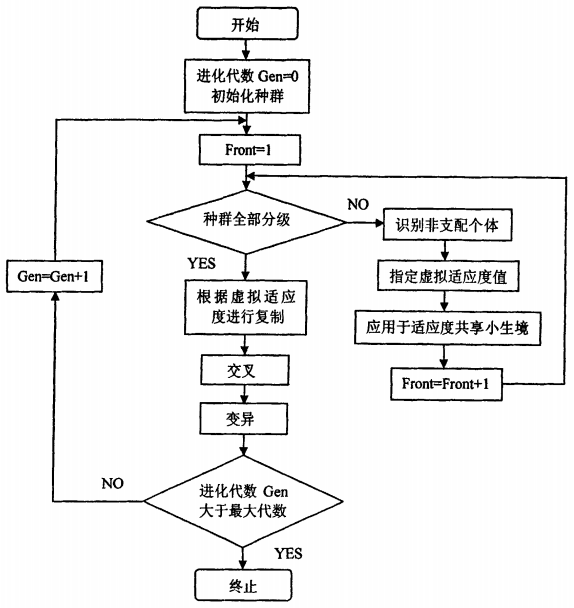• ## 多目标遗传算法及MATLAB代码

万次阅读 多人点赞 2020-01-03 23:12:49
需要求一个比较复杂的矩阵，一般方法解不出来，故尝试用多目标遗传算法（Multiobjective Genetic Algorithm）求解。不是专门做研究遗传算法的，根据自己需求进行了简单学习，并做如下笔记。 i 遗传算法理解 遗传...
需要求一个比较复杂的矩阵，一般方法解不出来，故尝试用多目标遗传算法（Multiobjective Genetic Algorithm）求解。不是专门做研究遗传算法的，根据自己需求进行了简单学习，并做如下笔记。
i 遗传算法理解
遗传算法属于一种搜索算法，通过不断试错，当结果满足提前设置精度时，停下来。理论上而言，只要计算时间足够长，计算能力足够大，穷举法就可以实现这一目标，并不需要这些听上去高大上的算法。关键问题是，如何快速收敛。遗传算法（Genetic algorithm）是一种从生物学获得灵感（往往运用最广的算法，核心都很简单，毕竟简单才方便推广），通过选择（selection）、交叉（crossover）和变异（Mutation）等操作让收敛速度加快，具体理论和操作前辈们早已论证，详见参考文献。类似算法还有模拟退火算法（Simulated Annealing）、粒子群算法（Paricle Swarm）等。

ii 基本概念解释
1. 概念
染色体（chromosome）：可能的解，编码化的解称为基因型（genetic type）解；实际的解称为表现型（pheno type）解
种群数（population size）：随机生成的初始解的个数；
适值函数（fitness function）： 评价染色体优劣性的函数，可以简单理解为与目标值的差异
目标函数（objective function）：限定函数，用于限制求解变量的函数
决策变量（decision variables）：所需要求解的变量，也就是求解问题的维数
遗传操作（genetic_operator）包括：
变异（mutation）：单个染色体中部分基因变化，保持多样性；变异率（mutaiton probability）变异操作的概率。
选择（selection）：选择出适应性高的染色体，进行下一代操作。
最大代数（maximum generaiton）：进化循环次数，最大迭代次数。

2. Pareto解或非支配解（non-dominated solution）
最大值问题，对于解X，
若不存在X1，使得F(X1)>F(X)，则X为弱Pareto最优解，此时F(X1)可以等于F(X)
若不存在X1，使得F(X1)>=F(X)，则X为Pareto最优解，也称非劣解

3. 多目标遗传算法种类
本文讨论非支配排序遗传算法（Non-dominated Sorting Genetic Algorithm II），即NSGA-II（参考文献【2】和《网络模型与多多目标遗传算法》P165）iii NSGA-II求解步骤和MATLAB代码
首先，根据自己的问题定义目标函数（objective_description_function）和评价函数（evaluate_objective），然后运行主函数即可。主函数由如下几个函数组成。
主函数  nsga_2 （i.e. 非支配排序遗传算法的求解步骤）
STEP 1: initialize_variables，随机生成染色体种群，随机解STEP 2: non_domination_sort_mod，解的非支配排序STEP 3: tournament_selection，精英策略的竞赛选择STEP 4: genetic_operator，基因操作STEP 5: replace_chromosome，替换第一代染色体，进入下一次迭代

MATLAB代码
Aravind Seshadri (2020). NSGA - II: A multi-objective optimization algorithm, MATLAB Central File Exchange. Retrieved January 6, 2020.
CSDN joekepler 有将上述部分代码做中文注释
NSGA2 算法MATLAB完整代码 中文注释详解

【参考文献】
(入门了解)  超详细的遗传算法(Genetic Algorithm)解析  Kalyanmoy Deb, et al., A fast and Elitist multiobjective genetic algorithm: NSGA-II 雷英杰 等，MATLAB遗传算法工具箱及应用，西安电子科技大学出版社【其中很多函数已经被包装进GA，不能用了】[日] 玄光男，等，网络模型与多目标遗传算法，清华大学出版社[日] 玄光男，等，遗传算法与工程优化，清华大学出版社MATLAB Global Optimizaiton Toolbox NSGA2 算法MATLAB完整代码 中文注释详解 Aravind Seshadri (2020). NSGA - II: A multi-objective optimization algorithm, MATLAB Central File Exchange. Retrieved January 6, 2020.
展开全文遗传算法 NSGA
• 多目标遗传算法研究


第四章 多目标遗传算法研究
§4.1 多目标优化算法简介

实际的工程优化问题大多数是多目标优化问题，目标之间一般都是互相冲突的。多目标优化很早就得到了人们的重视，到目前已经发展了较多的求解多目标优化的方法。下面先介绍多目标优化最重要的关于非劣解以及非劣解集的定义。
定义1：若
（ 为多目标优化的可行域），不存在另一个可行点 ，使得 成立（ 为目标数），且其中至少有一个严格不等式成立，则称 是多目标优化的一个非劣解（Noninferior Solution）。所有非劣解构成的集叫非劣解集（Noninferior Set）。
一个多目标优化如果存在非劣解，往往存在无穷多个，形成非劣解集。在求解实际问题时，过多的非劣解是无法直接应用的。决策者只能选择令其最满意的一个非劣解作为最终解。求最终解主要有三类方法，一类是求非劣解的生成法，即先求出大量的非劣解，构成非劣解的一个子集，然后按照决策者的意图找出最终解，另一类为交互法，不先求出很多的非劣解，而是通过分析者与决策者对话的方式，逐步求出最终解。最后一类是事先要求决策者提供目标之间的相对重要程度，算法以此为依据，将多目标问题转化为单目标问题进行求解，该类方法也可以被认为是第一类方法的一个子方法，该类方法的难点在于，如何得到决策者真实的权重信息，本章将提出一种基于模糊逻辑的，能比较好反映决策者权重的多目标遗传算法。
生成法主要有加权法﹑约束法﹑加权法和约束法结合的混合法以及多目标遗传算法。而交互法主要有用于求解线性约束多目标优化的Geoffrion法﹑求解线性多目标优化的逐步法（STEM）和Zionts-Wallenius方法以及代替价值交换法。
作者认为相对而言生成法对决策者更有吸引力，首先目前没有比较好的多目标非线性优化的交互法，其次在只给决策者有限信息的前提下，往往要求决策者回答一些似是而非的问题，决策者在交互过程中将会很被动，也就是说交互法在某种程度上将问题的矛盾转嫁给了决策者。如果我们能求得非劣解的一个较好的近似解集，决策者就有了一个对问题比较全面的认识，从而能更好地进行决策和折衷。
生成法中，常用的加权法有其固有的缺点，对于非劣解集的某些区域不可能求出。如图4.1就是一个两个目标的例子，箭头所指非劣解曲线凹的部分无法用加权法求出，出现该现象的原因是，加权法实际上是优化各个目标函数正线性组合而成的单个目标。即采用正加权系数计算的单目标最优必然是非劣解，而某些非劣解可能找不到一组正加权系数来进行求解。采用约束法可以避免该现象，但是计算代价过大，且过程很繁杂，应用前景也不乐观。利用多目标遗传算法求解非劣解集是最近几年新出现的一种求解思路，目前还处在研究

图4.1 加权法无法求出全部非劣解的例子
的初始阶段，但是初步的计算结果非常令人振奋，故本章另一个研究重点就放在了多目标非劣解集算法的研究上。
§4.2基于模糊逻辑的多目标遗传算法
§4.2.1 模糊逻辑简介
模糊逻辑（Fuzzy Logic）是一种新型的分类方法（分类是集合论的基本概念之一）。模糊逻辑模仿人类的智慧，引入隶属度的概念，描述介于“真”与“假”中间的过渡过程。在模糊逻辑中，事件不以集合的极限值分类，而是给每一个元素赋予一个介于0和1之间的实数，描述其属于一个集合的强度。具体的介绍可以参考文献【116】。
§4.2.2 基于模糊逻辑的多目标遗传算法的思路和具体实现
通过前面的介绍，我们知道采用模糊逻辑是一种很好的反应决策者主观意愿的工具。可以用模糊逻辑的方法反应决策者对于各个目标之间重要性的“权衡”信息。众所周知，遗传算法是依据个体的适应度进行，可以认为适应度就是决策者对于个体的综合评价，因而可以依据模糊逻辑的方法，直接构造决策者对于遗传个体的适应度的取值，即决策者对个体的综合评价。并以此作为遗传进化的依据和动力。下面是初步的算法步骤：
（1）分别求出各单目标的最优解；
（2）以（1）中的单目标的最优解和决策者协商，给出各个目标满意度的隶属函数；
（3）通过模糊逻辑表达决策者的想法，将各目标的满意度和个体的适应度联系起来；
（4）以（3）定义的适应度为基础采用遗传算法进行求解；
之所以首先求解各个单目标的最优解，是希望能给决策者一个比较清晰的概念，即如果单独优化某个目标，可能的最优解是多少。有了这个信息，决策者才能给出令人置信的关于不同目标的满意度的隶属曲线。如果求解单目标优化的难度很大，也可以给出对单目标最优的一个估计值。
具体的计算方法和模糊控制的计算方法基本相同，也分为模糊化（Fuzzification）﹑模糊推理（Fuzzy-Inference）和去模糊化（Defuzzification）三部分组成。在作者算法中，模糊推理采用的是最大最小法，而去模糊化采用的是面积重心法。详见参考文献【116】。
下面以十杆桁架双目标优化为例详细叙述算法过程。
参考图3.4，位移目标是指节点1﹑2﹑3﹑4的最大 向位移最小。这里最大 向位移指的是绝对值最大。
这样我们就有了两个目标，即同时极小化位移和重量，可以建立比较简单的模糊逻辑如下：
（1）如果重量轻并且位移小那么设计的适应度高；
显然上式同时对两个目标都提出了要求。而如果我们只关心重量则可以建立如下的模糊逻辑：
（2）如果重量轻不管位移大或小设计的适应度都应高；
我们可以将模糊逻辑表示得更加精细，更符合决策者实际的想法：
（3-1）如果重量轻而且位移小那么设计的适应度很高；
（3-2）如果重量轻而且位移中等那么设计的适应度高；
（3-3）如果重量轻而且位移大那么设计的适应度一般；
（3-4）如果重量中等而且位移小那么设计的适应度高；
（3-5）如果重量中等而且位移中等那么设计的适应度中等；
（3-6）如果重量中等而且位移大那么设计的适应度低；
（3-7）如果重量大而且位移小那么设计的适应度中等；
（3-8）如果重量大而且位移中等那么设计的适应度低；
（3-9）如果重量大而且位移大那么设计的适应度很低；
我们可以建立相应的表格表示以上逻辑：
重量 位移 → 设计适应度 Good Good → Very High Good Normal → High Good Bad → Normal Normal Good → High Normal Normal → Normal Normal Bad → Low Bad Good → Normal Bad Normal → Low Bad Bad → Very Low
表4.1 模糊逻辑表
采用模糊逻辑的方法实际上将目标函数映射到决策者的效用函数上，一般来说决策者的效用函数的精确描述是很困难的，目前多目标优化研究的一个重要领域就是如何合理构造决策者的效用函数。但是要决策者用带有模糊性的语言对自己的偏好加以描述却比较容易的。采用模糊逻辑的另外一个优点就是，决策者可以直观地观察到自己如果改变了模糊逻辑后，决策的变化。即求出的解始终是与决策者的真实想法接近的。在非劣解集范围较大的情况下，采用模糊逻辑可能是唯一实际可行的办法。
§4.2.3 算例
在上一章已经求得十杆问题的最轻重量为1598.93lb，相应的最大位移为7.171639 ，我们也可以求得最小位移为0.977492 ，相应的最大重量为15349.4496 。下面是是位移最小和重量最轻的设计点的比较。
位移最小设计（ ） 最轻设计（ ） 杆1 40.0000 7.9396 杆2 40.0000 0.1 杆3 40.0000 8.0956 杆4 40.0000 3.9613 杆5 0.1 0.1 杆6 40.0000 0.1 杆7 40.0000 5.7554 杆8 40.0000 5.5994 杆9 40.0000 5.5994 杆10 40.0000 0.1 重量（ ） 15349.4496 1598.93 位移（ ） 0.977492 7.171639
表4.2
从上表可以很明显地看出，位移和重量是两个互相冲突的目标。
在已知单目标极值的情况下我们采取一简单的模糊逻辑来确定个体的适应度，如果重量轻并且位移小那么适应度高。
为简单起见，假定重量轻的隶属函数为线性的，如图4.2所示。

1

1598.93

15349.4496

重量

图4.2 重量轻的隶属函数
假定位移小的隶属函数也为线性，如图4.4所示。
个体适应度的取值范围定义为[0,1]，适应度高的隶属函数也假定为线性，如图4.3所示。
群体规模取100，代数为500代，复制概率为0.2，变异概率为0.01，随机运行十次得到如下结果：
随机运行十次得结果如下：
重量（ ） 位移（ ） 4567.742671 2.288459 4645.949119 2.349095 4688.605046 2.378970 4714.601695 2.381532 4887.722703 2.460164


展开全文• ## 多目标遗传算法NSGA

万次阅读 多人点赞 2017-05-01 22:19:47
多目标遗传算法NSGA因所读的一篇论文中，为了解决目标的最优解问题，作者使用了一种称为NSGA-II(Improved Non-dominated Sorting Genetic Algorithm)的遗传算法，花了两天时间了解下，此为何物。其中NSGA以及NSGA-...
多目标遗传算法NSGA
因所读的一篇论文中，为了解决多目标的最优解问题，作者使用了一种称为NSGA-II(Improved Non-dominated Sorting Genetic Algorithm)的遗传算法，花了两天时间了解下，此为何物。其中NSGA以及NSGA-II的原理说明内容大部分取自2008年李莉的硕士论文《基于遗传算法的多目标寻优策略的应用研究》，故将此文定为转载。
首先需要了解一种称之为‘dominate’的关系：  设一个最大化目标函数为

F(x)=(F1(x),F2(x),...,Fk(x))
$F(x) = (F_1(x), F_2(x), ..., F_k(x))$, 因为是一个多目标最优的问题，所以这里的目标数量

k≥2
$k \ge 2$。假定

x0
$x_0$,

x1
$x_1$是解空间

X
$X$中的两个解。如果∃i∈[1,k]$\exists i \in [1,k]$使得

Fi(x0)>Fi(x1)
$F_i(x_0) > F_i(x_1)$成立，并且

∀i∈[1,k]
$\forall i \in [1, k]$时，

Fi(x0)≥Fi(x1)
$F_i(x_0) \ge F_i(x_1)$也成立，那么就称解

x0
$x_0$占优(dominate)

x1
$x_1$。如果在所有的解空间

X
$X$中找不到其他能占优x0$x_0$的解，那么我们称解

x0
$x_0$是一个efficient solution。该

x0
$x_0$在空间中对应的点，称为 non-dominated point。个人感觉efficient solution其实就是一个Pareto最优解，即，不可能在使得至少一个人收益变得更好情况下而保证其他人的收益不变差。  一般而言，在多目标优化的问题里， efficient solution往往不是唯一的，那么所有efficient solutions的集合，我们称为efficient set。相对应地，所有non-dominated points组成的点集，称为Pareto front(帕累托前沿)。
题外话：一般多目标规划问题，其实都可以建模为找Pareto 最优解的问题。
在一个庞大的解空间中找出所有的Pareto解(Pareto front)是一个NP-hard问题，因此一些有意思启发式算法就诞生了，而本文这里所讲的遗传算法(Genetic Algorithm)就是其中之一。
NSGA(Non-dominated Sorting Genetic Algorithm)
NSGA非支配排序遗传算法就是一种以基本遗传算法为基础的多目标寻优策略，因为其在多目标寻优领域的优势，成为人们的研究热点。
下面将简要说明NSGA的原理[]。  NSGA主要由三部分构成，分别为：
种群分层  假定寻找最大化目标函数为

F(x)=(F1(x),F2(x),...,Fm(x))
$F(x) = (F_1(x), F_2(x), ..., F_m(x))$，种群规模为

n
$n$。
(1)设i=1$i=1$；  (2)对于所有的

j=1，2，…，n
$j=1，2，…，n$且

j≠i
$j \ne i$，按照以上定义比较个体

xi
$x_i$和个体

xj
$x_j$之间的支  配(dominate)与非支配(non-donimated)关系；  (3)如果不存在任何一个个体

xj
$x_j$优于

xi
$x_i$，则

xi
$x_i$标记为非支配个体；  (4)令

i=1+1
$i=1+1$，转到步骤(2)，直到找到所有的非支配个体。  通过上述步骤得到的非支配个体集是种群的第一级非支配层，然后，忽略这些已经  标记的非支配个体(即这些个体不再进行下一轮比较)，再遵循步骤(1)一(4)，就会得到第二  级非支配层。依此类推，直到整个种群被分层。共享小生境技术  为了在演化过程中保持群体的多样性，NSGA中引入了共享小生境技术。  假设第

p
$p$级非支配层上有np$n_p$个个体，每个个体的虚拟适应度值为

fp
$f_p$。  (1)算出同属于一个非支配层的个体

xi
$x_i$和个体

xj
$x_j$的欧几里得距离：

dij=∑l=1l=m(Fl(xi)−Fl(xj)Ful−Fdl)2−−−−−−−−−−−−−−−−−⎷

\begin{equation}
d_{ij} = \sqrt{\sum_{l=1}^{l=m}(\frac{F_l(x_i)-F_l(x_j)}{F_l^u-F_l^d})^2}
\end{equation}  其中

m
$m$目标个数，Ful,Fdl$F_l^u,F_l^d$分别为

Fl
$F_l$的上界和下界。  (2)共享函数(Sharing Function)是表示两个个体间关系密切程度的函数，两个个体

xi
$x_i$和

xj
$x_j$间的共享函数

sh(dij)
$sh(d_{ij})$:

sh(dij)=⎧⎩⎨⎪⎪1−(dijσshare)α0,dij≤σsharedij>σshare

\begin{eqnarray*}
sh(d_{ij}) = \left\{
\begin{array}{ll}
1 - (\frac{d_{ij}}{\sigma_{share}})^{\alpha} & , d_{ij} \le  \sigma_{share}    \\
0 & d_{ij} >  \sigma_{share}
\end{array}
\right.
\end{eqnarray*}

σshare
$\sigma_{share}$的值表示了

xi
$x_i$与

xj
$x_j$群体的相似度。

dij
$d_{ij}$表示个体

xi
$x_i$与

xj
$x_j$间的欧式距离。

α
$\alpha$用于对

sh(dij)
$sh(d_{ij})$的调整。  由此可见：

sh(dij)
$sh(d_{ij})$越大表明二者关系密切，即相似度高  (3)然后我们计算出节点

i
$i$与其他所有节点的累积相似度，称为共享度ci$c_i$:

ci=∑j=1npsh(dij),i=1,2,...,np

\begin{equation}
c_i = \sum_{j=1}^{n_p}sh(d_{ij}), i = 1,2,...,n_p
\end{equation}  (4)计算出个体

xi
$x_i$的共享适应度值:

f′p(xi)=fp(xi)/ci

\begin{equation}
f_p^{'}(x_i) = f_p(x_i)/c_i
\end{equation}  同理我们可以计算出所有个体在小生境条件下的适应度，从而提高了种群在演化时的多样性，因为相似度高的种群，其适应度会得到适当地减小。
整体NSGA工作流程如下图所示(至于遗传算法的具体内容，如果以后接触到，再做详细地了解)：展开全文• 寻找非劣解集合是遗传算法求解目标优化问题的目标, 而标准的遗传算法收敛性分析方法对多目标遗传算法的分析 并不合适。本文利用有限马尔科夫链给出了遗传算法求解目标优化问题的两个收敛性定义, 并给出了一个...
• %% 遗传算法求解多目标优化案例 %% 将原多目标函数改写为f1=(x^2+y^2)/4;f2=x(1-y)+10; % 运用线性叠加法，F=a*f1(x)+b*f2(x) ,a+b=1 % 总目标函数改写为 f=0.6*(x^2+y^2)/4+0.4*(x*(1-y)+10); popse=100...
function MultiGA()
%% 运行此程序之前先安装谢尔菲德遗传算法工具箱。 %% 遗传算法求解多目标优化案例 %% 将原多目标函数改写为f1=(x^2+y^2)/4;f2=x(1-y)+10; % 运用线性叠加法，F=a*f1(x)+b*f2(x)  ,a+b=1 % 总目标函数改写为  f=0.6*(x^2+y^2)/4+0.4*(x*(1-y)+10);   popse=100;  % 种群数目 maxgen=50;  % 最大迭代次数 preci=20;   % 编码长度 gap=0.95;   % 代沟大小 px=0.7;     % 交叉概率 pm=0.01;    %变异概率 lbx=1;      % 变量下界 lby=1; ubx=4;      % 变量上界 uby=2; trace=zeros(3,maxgen);  % 定义寻优结果的初始值 FieldD=[preci preci;lbx lby;ubx uby;1 1;0 0;1 1;1 1]; % 区域描述器 chrom=crtbp(popse,preci*2);  % 创建随机种群 % 优化 gen=0; pop=bs2rv(chrom,FieldD);    % 进制转换 X=pop(:,1);Y=pop(:,2); objv=0.15*(X.^2+Y.^2)+0.4*(X.*(1-Y)+10);  % 目标函数 while gen < maxgen     fitnv=ranking(-objv);     selch=select('sus',chrom,fitnv,gap);    % 选择 ‘sus’表示为随机遍历抽样     selch=recombin('xovmp',selch,px);       % 重组  ‘xovmp’表示多点交叉     selch=mut(selch,pm);     pop=bs2rv(selch,FieldD);                 % 子代个体进行进制转换     X=pop(:,1);Y=pop(:,2);     objvsel=0.15*(X.^2+Y.^2)+0.4*(X.*(1-Y)+10);     [chrom,objv]=reins(chrom,selch,1,1,objv,objvsel);     pop=bs2rv(chrom,FieldD);     gen=gen+1;     [Y,I]=max(objv);     trace(1:2,gen)=pop(I,:);     trace(3,gen)=Y; end %% 画出求解结果 figure(1) plot3(trace(1,:),trace(2,:),trace(3,:),'b');  % 画出每代最优点 xlabel('x') ylabel('y') zlabel('f') figure(2) plot(1:maxgen,trace(3,:));   xlabel('迭代次数') ylabel('最优解') bestz=trace(3,end); bestX=trace(1,end); bestY=trace(2,end); fprintf(['最优解：\n X=',num2str(bestX),'\n Y=',num2str(bestY),'\n z=',num2str(bestz)])
展开全文• ## 多目标遗传算法优化

万次阅读 热门讨论 2017-03-31 20:29:37
%% 遗传算法求解多目标优化案例 %% 将原多目标函数改写为f1=(x^2+y^2)/4;f2=x(1-y)+10; % 运用线性叠加法，F=a*f1(x)+b*f2(x) ,a+b=1 % 总目标函数改写为 f=0.6*(x^2+y^2)/4+0.4*(x*(1-y)+10); popse=100; % ...
• 对于单目标优化问题，一般的遗传算法可以较为简单的得到较...这时候，如何寻找合适的适应度函数便成解决多目标遗传算法的关键。如今，相关的算法已经有很种了。包括妥协算法（compromise approach），GWASF-GA，S...
• 遗传算法是用于无约束优化问题的单目标优化技术。 GA 有多种实现方式，其中一种采用 SBX 交叉和多项式变异。 该代码源自 Arvind Sheshadari  对 NSGA-II 的多目标实现。 笔记： （i）与其他计算智能技术不同，...matlab
• 对任意目标函数的优化问题转化成两个目标函数的优化问题，并对转化后的优化问题设计了遗传算法，同时把均匀性分布指标函数引入算法的变异操作中，用于自适应地调节搜索向Pareto最优解集移动和更好地获得解的均匀...
• 基于这样一种应用需求，单目标遗传算法很明显已经不能满足工程实践的要求了，所以需要开拓多目标的优化算法，多目标遗传算法就是在这样的背景下，好吧，是我自己需要进行多目标的优化，跟大背景没有太大的关联。...python
• 遗传算法 建立GeneticAlgorithm.py import numpy as np from GAIndividual import GAIndividual import random import copy import matplotlib.pyplot as plt class GeneticAlgorithm: ''' The class for....
• 用于求解多目标优化问题 object1 和object 2有一定的竞争关系 得到的解是一组解，称为帕累托前沿 pareto front options = optimoptions('gamultiobj','PlotFcn',... {@gaplotpareto},'UseParallel',true); func=@...
• 多目标优化系列： MOP_1. 多目标优化的相关基本概念 MOP_2. 非支配排序遗传算法 —（NSGA、...1995年，Srinivas和Deb提出了非支配排序遗传算法(Non-dominatedSorting Genetic Algorithms，NSGA)。这是一种基于P...NSGA
• （背包问题）：背包只能容得下一定重量b的物品，物品有m种，每种物品有自己的重量w(i)和价值v(i)，从这些物品中选择装入背包，是背包不超过重量b，但价值又要最大。... 遗传算法作经典的人工智能算法，可以很c++ 背包问题
• 多目标优化求解思路二.NSGA-II算法解析1.快速非支配排序(Fast non-dominated sort)2.拥挤距离计算(Crowding distance assignment)3.精英保存策略(Elitism)三.NSGA-II算法实现1.测试函数 一.多目标优化简介 1.多目标...NSGA-II
• 本文档是博客《多目标快速非支配排序遗传算法-NSGA-II》中所需要拜读的参考文献论文，包含了NSGA,NSGA-II，NGPM手册及Deb K 所撰写的与NSGA相关的部分论文，
• 遗传算法(Genetic Algorithm)中的基因，并不一定真实地反映了待求解问题的本质，因此各个基因之间未必就相互独立，如果只是简单地进行杂交，很可能把较好的组合给破坏了，这样就没有达到累积较好基因的目的，
• @多目标优化问题和遗传算法学习笔记 多目标优化问题和遗传算法学习笔记 你好！ 这是你第一次使用 Markdown编辑器 所展示的欢迎页。如果你想学习如何使用Markdown编辑器, 可以仔细阅读这篇文章，了解一下Markdown的...matlab 自然语言处理
• ## 遗传算法

万次阅读 多人点赞 2019-04-06 21:41:47
使用遗传算法求解峰函数的最大值，是我的一项课程作业，做完之后，顺便把文档整理出来做个记录。全部内容如下： 1、问题描述 编程实现遗传算法，并求解峰函数的最大值。峰函数的表达式如下所示： 用MATLAB...C语言
• 第九章 基于遗传算法多目标最优化算法基础理论pareto最优解多目标优化NSGA一II算法的基本思想(1) 基本原理(2) 算法流程(3) 算法缺陷2. 带精英策略的非支配排序的遗传算法(NSGA-II) 基础理论 pareto最优解 带精英...python
• 多目标优化算法，非支配的精英策略遗传算法：NSGA-II 1.算法简介 NSGA-II算法特点：快速非支配排序算法、精英保留策略、拥挤度分配策略。 相比于NSGA的优势： 排序算法的时间复杂度 O(MN2)O(MN^2)O(MN2) ...NSGA-II Matlab
• 之前学习了遗传算法对单目标函数的最优值求解，对于多目标问题。或者说是变量参数的求解问题，我想再研究一下。正好，我也想改进一下之前的代码架构。不得不说，之前的代码是面向过程的架构，完全没有体现出python...
• 新高考模式下遗传算法在排课问题中的应用 背景： 随着新高考改革在各个省份的推行，提出了“3+3模式”，即高中阶段的学生，不再区分文理科目，学生可以自主的从政治、历史、地理、物理、化学、生物和技术这7门课程里...排课问题
• 网上有很博客讲解遗传算法，但是大都只是“点到即止”，虽然给了一些代码实现，但也是“浅尝辄止”，没能很好地帮助大家进行扩展应用，抑或是进行深入的研究。 这是我的开篇之作~之前没有写博客的习惯，一般是将...约束优化 geatpy GA工具箱...